#### Supported by: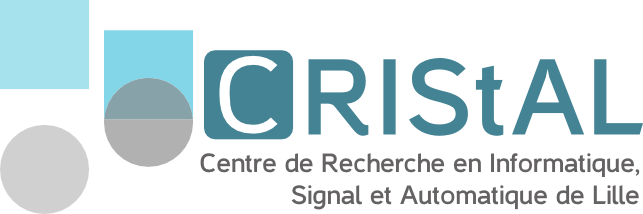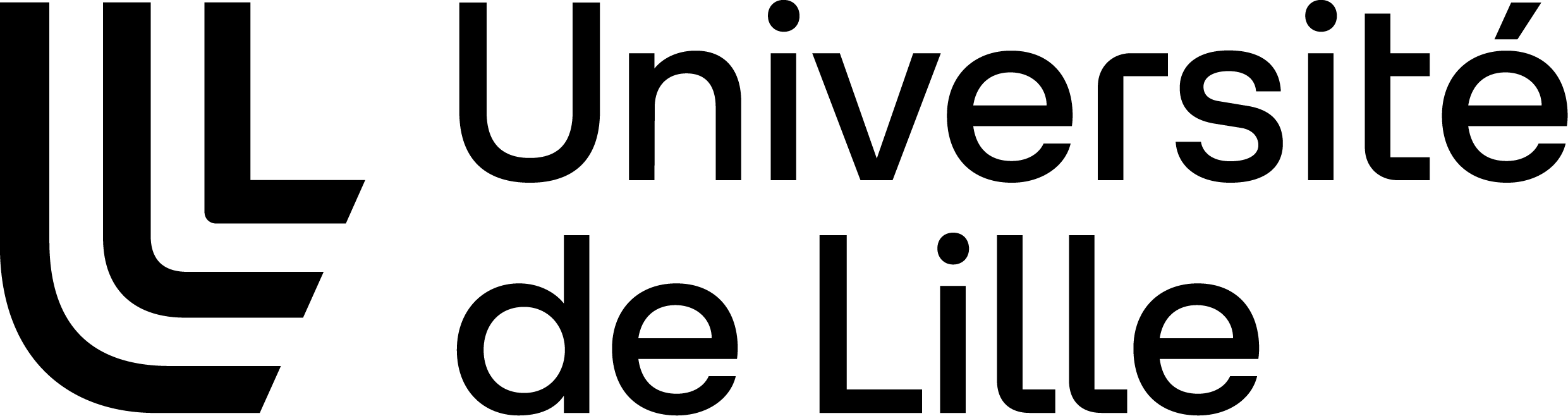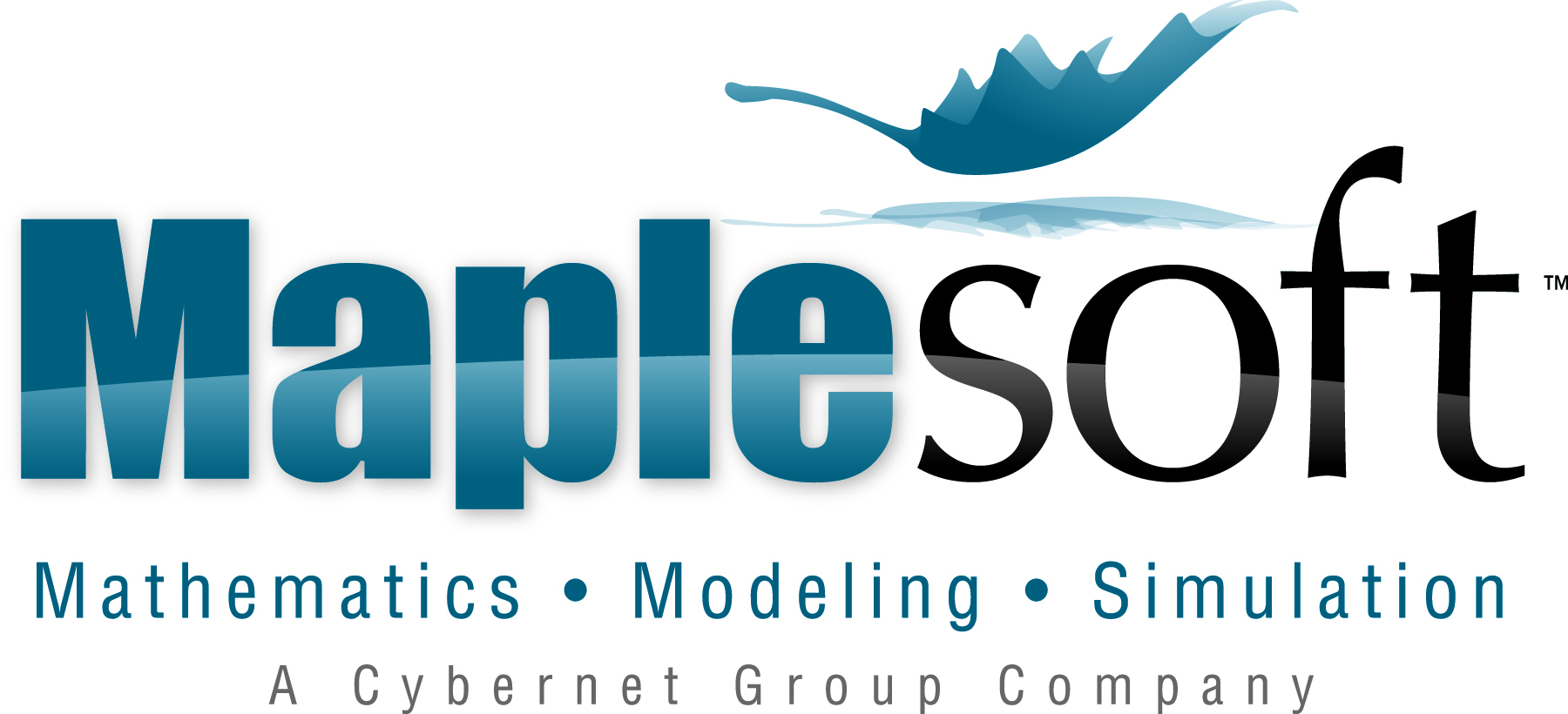# Invited Speakers

## Titles and Abstracts

Mioara Joldes
CNRS, LAAS, France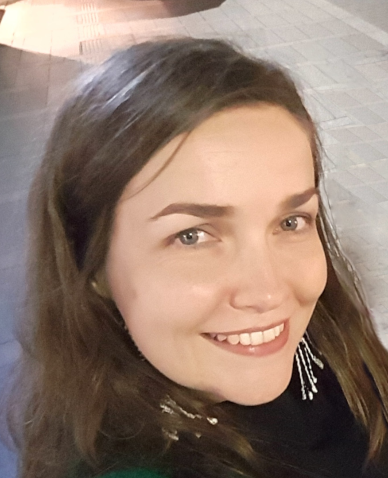### Validated numerics: algorithms and practical applications in aerospace

Abstract: In various fields, ranging from aerospace engineering or robotics to computer-assisted mathematical proofs, fast and precise computations are essential. Validated (sometimes called rigorous as well) computing is a relatively recent field, developed in the last 20 years, which uses numerical computations, yet is able to provide rigorous mathematical statements about the obtained result, such as guaranteed and reasonably tight error bounds. This area of research deals with problems that cannot or are difficult and costly in time to be solved by traditional mathematical methods, like problems that have a large search space, problems for which closed forms given by symbolic computations are not available or too difficult to obtain, or problems in nonlinear analysis.
In this talk, we provide an introduction to several computing methods and algorithms developed based on the theory of set-valued analysis (in specific function spaces) as well as by combining symbolic and numerical computations. These techniques are illustrated with some applications related to the efficient finite precision evaluation of numerical functions (some of which appear in practical space mission analysis and design).

Joris van der Hoeven
CNRS, LIX, France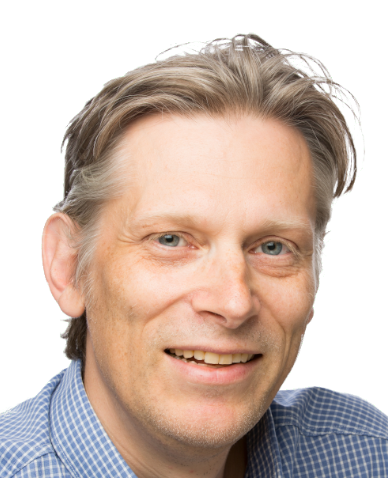### On the complexity of symbolic computation

Abstract: How fast can we perform basic operations in symbolic computation and how does this impact the complexity for solving more elaborate problems? In our talk we will survey computer algebra from this complexity perspective, while considering both theoretical and practical aspects.

Avi Wigderson
Institute for Advance Study, Princeton, USA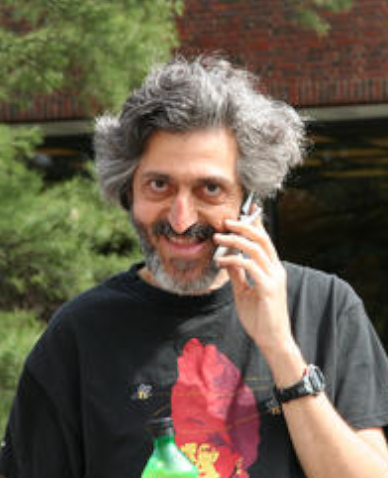### Non-commutative Optimization: where algebra, analysis and algorithms meet

Abstract: This talk aims to summarize a project I was involved in during the past 5 years, with the hope of explaining our most complete understanding so far, as well as challenges and open problems. The main messages of this project are summarized below; I plan to describe, through examples, many of the concepts they refer to, and the evolution of ideas leading to them. No special background is assumed.
(1) The most basic tools of convex optimization in Euclidean space extend to a far more general setting of Riemannian manifolds that arise from the symmetries of non-commutative groups. We develop first-order and second-order algorithms, and analyze their performance in general. While proving convergence bounds requires heavy algebraic and analytic tools, convergence itself depends in an elegant way on natural "smoothness" parameters, in analogy with the Euclidean (commutative) case.
(2) These algorithms give exponential (or better) improvements in run-time for solving algorithmic many problems across CS, Math and Physics. In particular, these include problems in algebra (e.g. testing rational identities in non-commutative variables), in analysis (testing the feasibility and tightness of Brascamp-Lieb inequalities), in quantum information theory (to the quantum marginals problem), in algebraic geometry (to computing Kronecker coefficients), in computational complexity (to derandomizing new special cases of the PoIynomial Identity Testing problem) and in optimization (to testing membership in large, implicitly described polytopes).
(3) The focus on symmetries exposes old and reveals new relations between the problems above, and between analysis, algebra and algorithms. Essentially, they are all membership problems in null cones and moment polytopes of natural group actions on natural spaces. Invariant theory, which studies such group actions, plays an essential role in this development. In particular, a beautiful non-commutative duality theory (expending linear programming duality in the commutative case), and notions of geodesic convexity (extending the Euclidean one) and moment maps (extending the Euclidean gradient) are central to the algorithms and their analysis. Interestingly, most algorithms in invariant theory are symbolic/algebraic, and these new numeric/analytic algorithms proposed here often significantly improve on them.

Based on joint works with Zeyuan Allen-Zhu, Peter Bürgisser, Cole Franks, Ankit Garg, Leonid Gurvits, Pavel Hrubes, Yuanzhi Li, Visu Makam, Rafael Oliveira and Michael Walter.